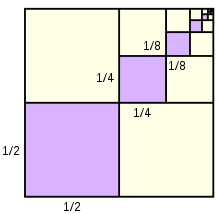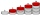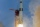# 10th term

What is the 10th term of the Arithmetic Progression if x1=4 and d=5?

x10 =  49

### Step-by-step explanation:Did you find an error or inaccuracy? Feel free to write us. Thank you!## Related math problems and questions:

• Arithmetic progression 2The 3rd term of an Arithmetic progression is ten more than the first term, while the fifth term is 15 more than the second term. Find the sum of the 8th and 15th terms of the Arithmetic progression if the 7th term is seven times the first term.
• HP - harmonic progression 2Compute the 16th term of the HP if the 6th and 11th term of the harmonic progression are 10 and 18 respectively.
• What is 10What is the 5th term, if the 8th term is 80 and common ratio r =1/2?
• HP - harmonic progressionDetermine the 10th term of the harmonic progression 6,4,3,…
• Missing term 2What is the missing term for the Geometric Progression (GP) 3, 15, 75,__, 1875?
• If the 3If the 6th term of a GP is 4 and the 10th is 4/81, find common ratio r.
• 15th term of APWhat is the 15th term; x1=1.5, d=4.5?
• Missing termWhat is the missing term in the sequence? _____, 13, 30, and 47?
• Find d 2Find d in an A. P. whose 5th term is 18 and 39th term is 120.
• HP - harmonic progressionDetermine the 8th term of the harmonic progression 2, 4/3, 1,…
• The sumThe sum of the first 10 members of the arithmetic sequence is 120. What will be the sum if the difference is reduced by 2?
• AP membersWhat is the value of x2, x3, x4, x5…of the terms (of arithmetic progression) when x1 = 8 and x6 = 20?
• Negative differenceFind four arithmetic progression members between 7 and -6.
• Common differenceThe 4th term of an arithmetic progression is 6. If the sum of the 8th and 9th terms is -72, find the common difference.
• A perineumA perineum string is 10% shorter than its original string. The first string is 24, what is the 9th string or term?
• Progression-12, 60, -300,1500 need next 2 numbers of pattern
• Investment 2Jack invested \$5000 in a 5-month term deposit at 4.7% p. A. . At the end of the 5-months, jack reinvested the maturity value from the first deposit into an 11-month term deposit at 7.3% p. A. What is the maturity value at the end of the second term deposi